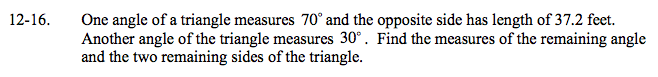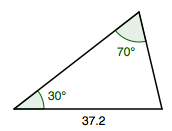### Home > A2C > Chapter 12 > Lesson 12.1.1 > Problem12-16

12-16.The sum of the angles in a triangle is 180°.

Use the Law of Sines to calculate the remaining side lengths.

$\frac{\text{sin}(70\degree)}{37.2}=\frac{\text{sin}(30\degree)}{x}$